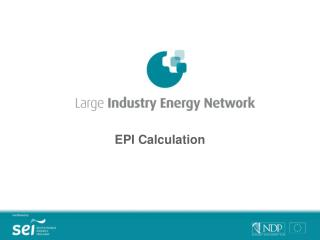DownloadDownload PresentationEPI Calculation

# EPI Calculation

Télécharger la présentation## EPI Calculation

- - - - - - - - - - - - - - - - - - - - - - - - - - - E N D - - - - - - - - - - - - - - - - - - - - - - - - - - -
##### Presentation Transcript

1. EPI Calculation

2. Contents • Energy Performance Index • Aggregate output selection • Weighted output example • EPI calculation • EPI report

3. Energy Performance Index • A company’s Energy Performance Index (EPI) is based on the ratio of annual primary energy consumption to aggregate product output • This output is developed individually by each company to reflect their unique mix of products and processes • For a member’s first year of joining the LIEN, their EPI is normalised to 100 • Subsequent improvements or deteriorations in energy performance are reflected in a decrease or increase of EPI

4. Aggregate Output Selection • For a single commodity manufacturer, the output unit is simply their base production unit • However, most firms have more than one product therefore an aggregate output must be selected • It is necessary to look at the relative energy intensity of each product and assign a weighting if appropriate • Must be a measure which can be meaningfully compared year-on-year

5. Weighted Output Example – Company XYZ

6. Energy per unit output If tonnes is used as output measure: • Energy/output 2001 = 60/1110 = 0.054 GWh/tonne = 54 MWh/tonne • Energy/output 2002 = 90/1170 = 0.077 GWh/tonne = 77 MWh/tonne

7. Weighted Output Example • It would not be representative to simply add the various quantities of Products A, B and C due to their different energy intensities • A more representative approach is to assign a weighting to each output relative to the amount of energy it takes to produce • In order to do this it must be possible to monitor the varying energy consumptions of each product e.g. meter each product line separately

8. Weighted Output Example – Company XYZ

9. Energy per unit output If weighted output is used as output measure: • Energy/output 2001 = 60/3000 = 0.02 GWh/wt output = 20 MWh/wt output • Energy/output 2002 = 90/4500 = 0.02 GWh/wt output = 20 MWh/wt output

10. Output selection Consider; • Weighted output may give a more representative indication if appropriate e.g. varying product mix and concentration • Value chosen much be comparable year-on-year e.g. is product line constantly changing

11. EPI calculation • Information is provided annually and must be reported in a manner that is confidential • LIEN members come from 13 different sectors with thousands or varying products • It is not possible to compare varying energy intensity units e.g. kWh/gallon, GWh/tonne, MWh/weighted production unit • Need to make comparison in energy intensity trends • Energy Performance Index developed

12. EPI calculation EPI = Energy x F Output where F is a factor used to set the EPI to 100 for a firm’s base year (i.e. the year they joined the LIEN or started reporting) F then is fixed for subsequent years

13. EPI example

14. EPI example

15. EPI Report • EPI report contains 5 pages of information specific to your site • Details trends in primary energy per unit of output since a company joined the LIEN • Gives information on estimated CO2 emissions as a result of energy use • Estimates how these specific emissions have changed from the previous year as a result of a firm’s energy performance

16. EPI Calculation Fiona Murray LIEN Project Manager